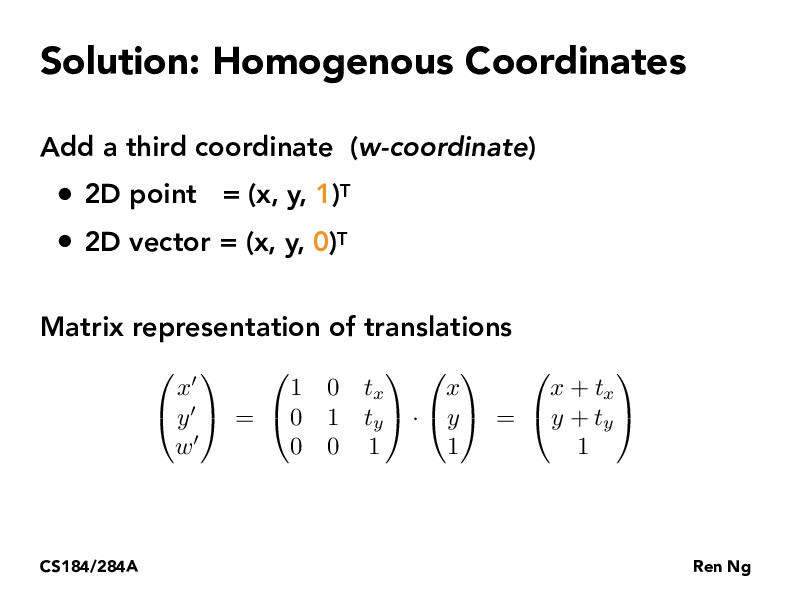Lecture 4: Transforms (23)bufudash

What is the point of homogenous coordinates? Since we can do this transformation in 2D, and homogenous coordinates just uses 3D. Does this just simply for the purpose of making higher dimension transformation simpler?andrewdotwang

I think the general idea is to use homogenous coordinates to more easily represent transformations through matrices and simplify the math formulas through the w-coordinate.jessicajyeh

As seen in the previous slides, transforms like rotation, scaling, are all linear transforms that we can accomplish in the form of x' = ax + by and y' = cx + by. For example, in 2D space, we can use a 2x2 matrix to represent rotation or scaling.

However with translations in particular, which are in the form x' = x + t_x and y' = y + t_y, we can't represent the addition of the t term using a 2x2 matrix. That's why we add an additional dimension - this dimension is a 'dummy' dimension in the sense that it doesn't actually represent 3D space, but it allows us to represent translations as matrix multiplications.

If we were in 3D space, then we'd add a fourth dimension for homogeneous coordinatesjamesyc23

Interesting idea, I would have thought to use 3D coordinates still and just add an affine term, but the idea of precomputing a bunch of transformations by multiplying matrices together makes this solution a bit more attractive.

You must be enrolled in the course to comment